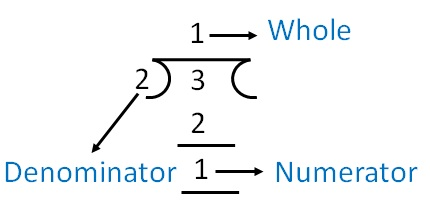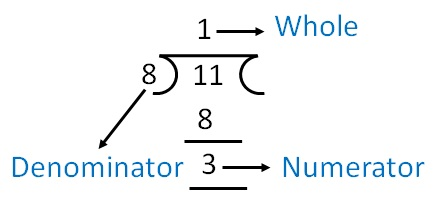### Convert 3/2 to mixed fraction

3/2So, 3/2 = 1 1/2

### Convert 11/8 into mixed fraction

11/811/8 = 1 3/8

1. Chapter 7 Class 6 Fractions
2. Concept wise
3. Proper, improper and mixed fraction

Transcript

Converting fraction to Mixed fraction Convert 3/2 to mixed fraction 3/2 So, 3/2 = 1 𝟏/𝟐 Convert 11/8 into mixed fraction 11/8 11/8 = 1 3/8 Convert 14/5 into mixed fraction 14/5 14/5 = 2 4/5